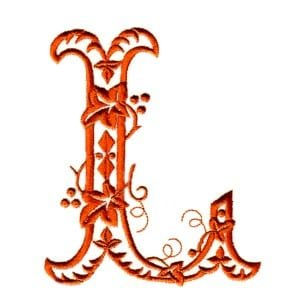How 9 Cheenta students ranked in top 100 in ISI and CMI Entrances?

# Dudeney Puzzle : A Tale from Pythagoras to Dehn

###et's travel back 2 Millenia to the Land of the Greeks where Plato, Pythagoras, Archimedes devoted their life to Math and Science to understand and enjoy nature. Math was a Puzzle to them and they enjoyed doing it as we do it now. Pythagoras gave birth to the beautiful proof of Pythagoras Theorem just using pictures.### This approach of dissection and rearrangement was not new to him as the tradition of these sort of puzzles can be traced back to Plato and also continued by Archimedes.### Square trisection: Three squares to One square### Two equal squares are turned into one square in fourteen pieces by subdivisions of the previous four pieces by Archimedes## Wallace–Bolyai–Gerwien theorem: A Polygon can be cut into a finite number of pieces and then by rotations, reflections and translations(isometric transformations) can be reassembled into another Polygon iff both the polygons have the same area. We call this equidecomposability of two polygons of the same area. [Actually, it is called equidissectabilty, but then it is equidecomposability]

### [A and B are scissors congruent if A can be cut into finitely pieces–each of which is homeomorphic to a disc and bounded by a curve of finite length–which can be rearranged to form B (ignoring boundaries).]## P $\rightarrow$ Q means Q $\rightarrow$ P## P $\rightarrow$ Q, Q $\rightarrow$ R means P $\rightarrow$ R## Triangle $\rightarrow$ A Rectangle (Not Predefined):## Rectangle  $\rightarrow$ A Square (Always fixed given an area):## 2 Squares $\rightarrow$ A Square (Always fixed given an area):# Knowledge Partner